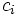# Artin-Tate lemma

View other indispensable lemmata
This article defines a result where the base ring (or one or more of the rings involved) is Noetherian
View more results involving Noetherianness or Read a survey article on applying Noetherianness

## Statement

Suppose$A, B, C$ are commutative unital rings, such that:

•$A$ is Noetherian
•$B$ and$C$ are$A$-algebras, with$B$ a subalgebra of$C$
•$C$ is finitely generated as an$A$-algebra (i.e.$C$ is an algebra of finite type over$A$)
•$C$ is finitely generated as a$B$-module (i.e.$C$ is finite as an algebra over$B$)

Then:$B$ is finitely generated as an$A$-algebra

## Explanation

The Artin-Tate lemma is related to the general philosophy that if an object is finitely generated, then any subobject of it is also finitely generated provided the subobject is almost the whole object. In our case,$C$ is finitely generated as an$A$-algebra, but it is not necessary that all subalgebras of$C$ are finitely generated as$A$-algebras. However, if the subalgebra is large enough inside$C$, i.e. it is not too deep, then it behaves like$C$, and we can try to show it is finitely generated.

An analogous result in group theory is the statement that any subgroup of finite index in a finitely generated group, is also finitely generated.

## Proof

### Find a finitely generated subalgebra of$B$

Pick a finite generating set$c_1, c_2, \ldots, c_r$ of$C$ as an algebra over$A$.

Since$C$ is finitely generated as a$B$-module, every element in$C$ is integral over$B$. In particular, every$c_i$ is integral over$B$. Choose a monic polynomial satisfied by$c_i$ over$B$ for each$i$, and consider the$A$-algebra$B_0$ generated by the coefficients of all such monic polynomials.

Clearly$B_0$ satisfies the following:

•$B_0$ is an$A$-subalgebra of$B$
•$B_0$ is finitely generated as an$A$-algebra
•$C$ is finitely generated as a$B_0$-algebra (since in fact$C$ is finitely generated as an$A$-algebra)
•$C$ is integral over$B_0$

### Going down

Since$C$ is both finitely generated as a$B_0$-algebra and integral over$B_0$, we see that$C$ is finitely generated as a$B_0$-module.

We now use the fact that$A$ is Noetherian, and$B_0$ is finitely generated as an$A$-algebra, to conclude that$B_0$ is Noetherian. Thus$C$ is a finitely generated module over a Noetherian ring$B_0$. Hence, the submodule$B$ is also finitely generated as a$B_0$-module. In particular,$B$ is finitely generated as a$B_0$-algebra.

### Transitivity

We know that$B_0$ is finitely generated as an$A$-algebra, and we just proved that$B$ is finitely generated as a$B_0$-algebra. Thus,$B$ is finitely generated as an$A$-algebra.